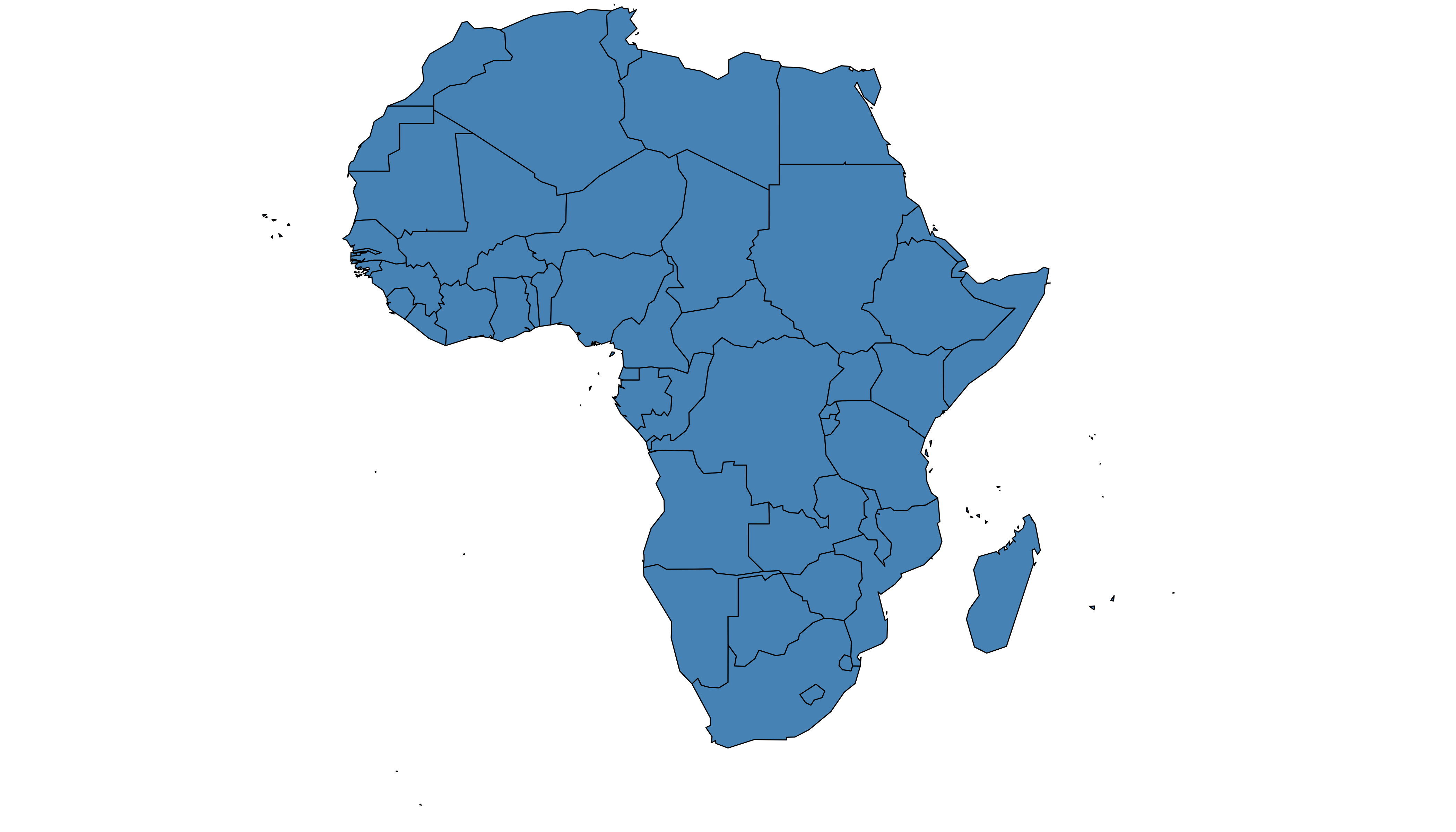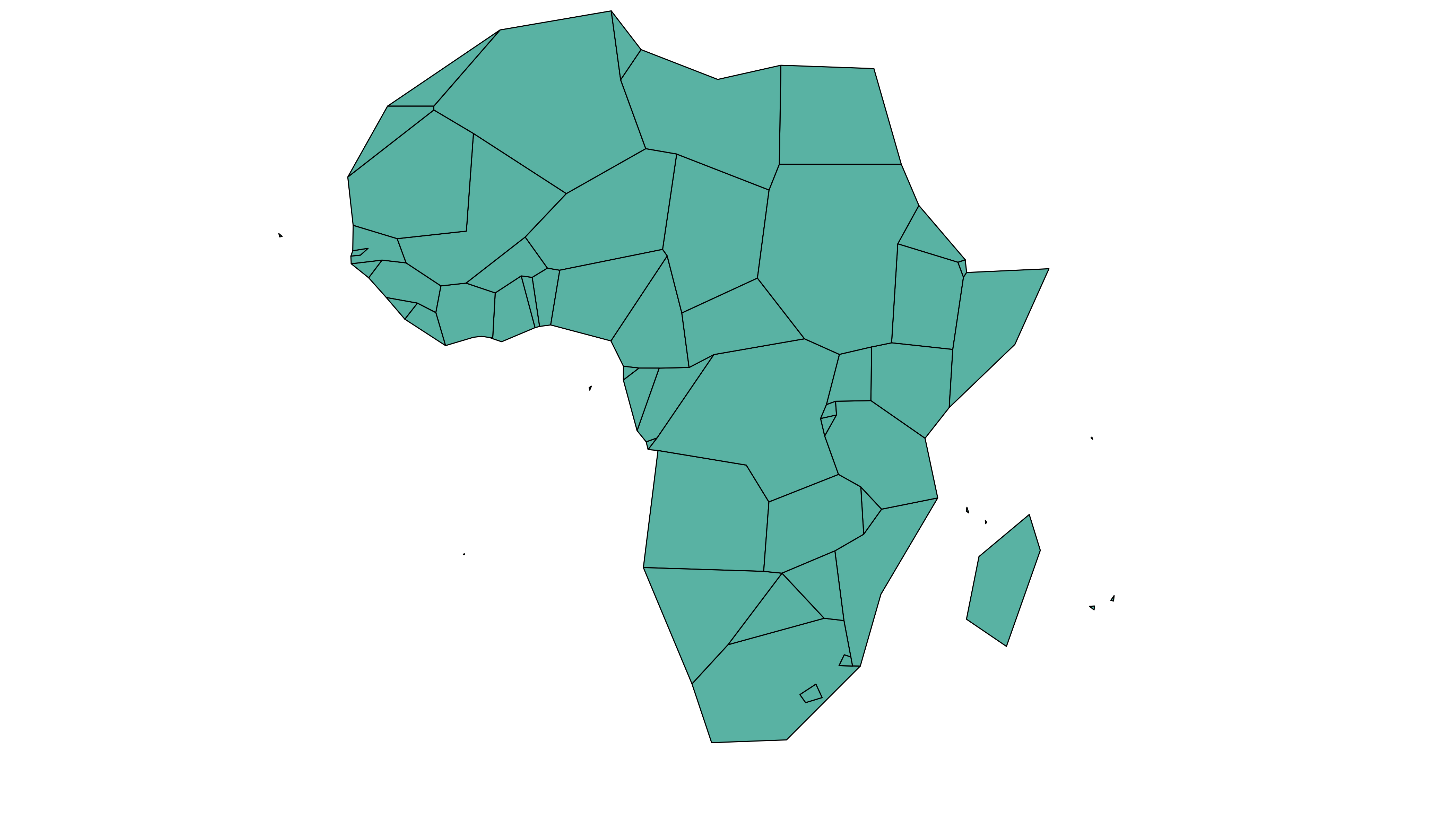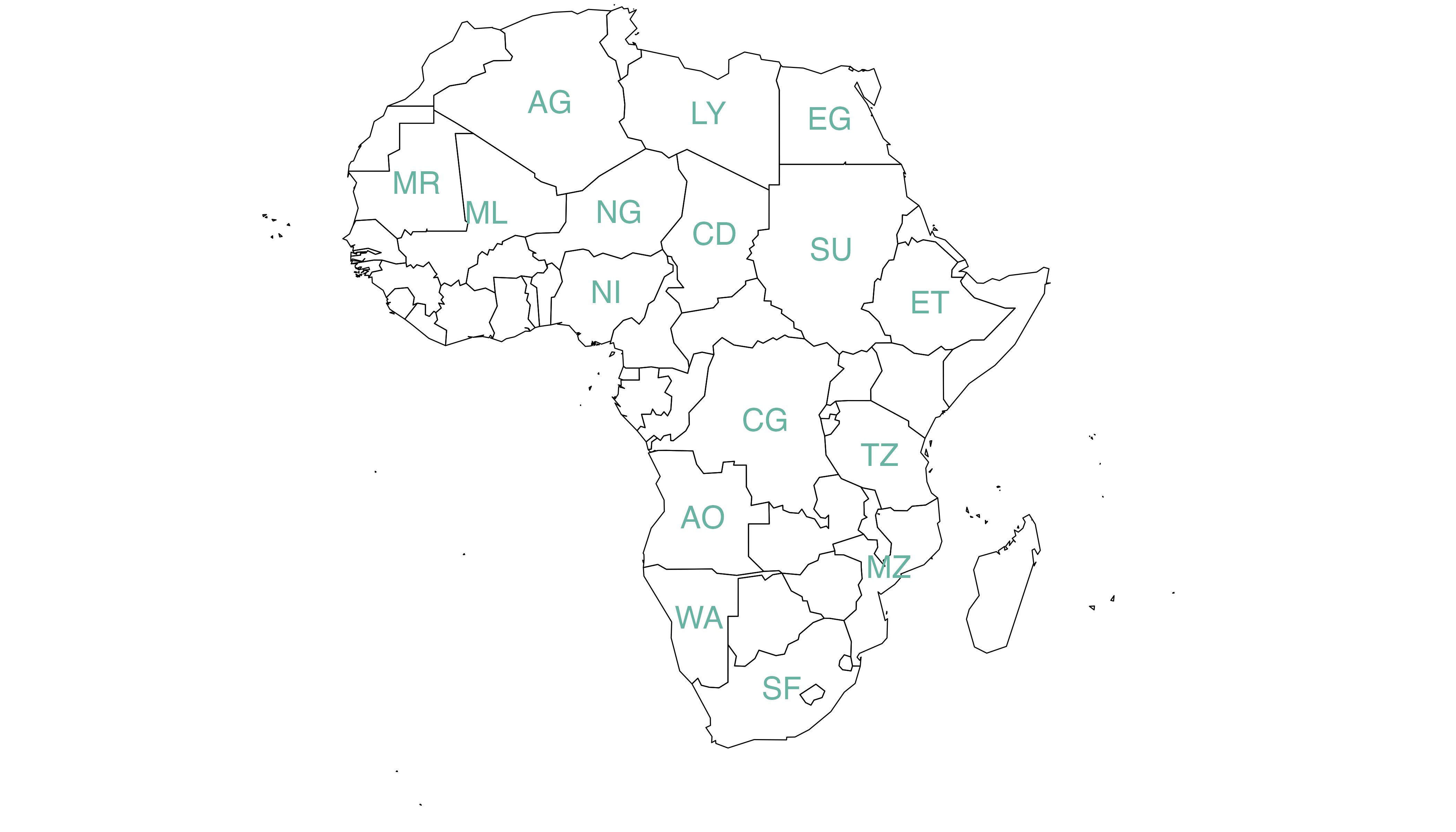Geospatial data manipulation in R

Map data are stored in a very specific geospatial format in R. This post describes the most common manipulations you may have to apply: selecting zone, simplifying the borders, and more.

Get a geospatial object

The region boundaries required to make maps are usually stored in geospatial objects. Those objects can come from shapefiles, geojson files or provided in a R package. See the map section for possibilities.

Let’s get a geospatial object from a shape file available here. This step is extensively described in this post in case you’re not familiar with it.

# Download the shapefile. (note that I store it in a folder called DATA. You have to change that if needed.)
# You now have it in your current working directory, have a look!

# Unzip this file. You can do it with R (as below), or clicking on the object you downloaded.
system("unzip DATA/world_shape_file.zip")
#  -- > You now have 4 files. One of these files is a .shp file! (TM_WORLD_BORDERS_SIMPL-0.3.shp)

And let’s load it in R

# Read this shape file with the rgdal library.
library(rgdal)
dsn= paste0(getwd(),"/DATA/world_shape_file/") ,
layer="TM_WORLD_BORDERS_SIMPL-0.3",
verbose=FALSE
)

# -- > Now you have a Spdf object (spatial polygon data frame). You can start doing maps!

Select a region

You can filter the geospatial object to plot only a subset of the regions. The following code keeps only Africa and plot it.# Keep only data concerning Africa
africa <- my_spdf[my_spdf@data\$REGION==2 , ]

# Plot africa
par(mar=c(0,0,0,0))
plot(africa , xlim=c(-20,60) , ylim=c(-40,35), col="steelblue", lwd=0.5 )

Simplify the geospatial object

It’s a common task to simplify the geospatial object. Basically, it decreases the border precision which results in a lighter object that will be plotted faster.

The rgeos package offers the gSimplify() function to makes the simplification. Play with the tol argument to control simplification rate.# Simplification with rgeos
library("rgeos")
africaSimple <- gSimplify(africa, tol = 4, topologyPreserve = TRUE)

# Plot it
par(mar=c(0,0,0,0))
plot(africaSimple , xlim=c(-20,60) , ylim=c(-40,35), col="#59b2a3", lwd=0.5 )

Compute region centroid

Another common task is to compute the centroid of each region to add labels. This is doable using the gCentroid() function of the rgeos package.library(rgeos)

# The gCentroid function computes the centroid of each region:
# gCentroid(africa, byid=TRUE)

# select big countries only
africaBig <- africa[which(africa@data\$AREA>75000), ]

# Small manipulation to put it in a dataframe:
centers <- cbind.data.frame(data.frame(gCentroid(africaBig, byid=TRUE), id=africaBig@data\$FIPS))

# Show it on the map?
par(mar=c(0,0,0,0))
plot(africa , xlim=c(-20,60) , ylim=c(-40,35), lwd=0.5 )
text(centers\$x, centers\$y, centers\$id, cex=.9, col="#69b3a2")

Related chart types

Contact

This document is a work by Yan Holtz. Any feedback is highly encouraged. You can fill an issue on Github, drop me a message on Twitter, or send an email pasting yan.holtz.data with gmail.com.